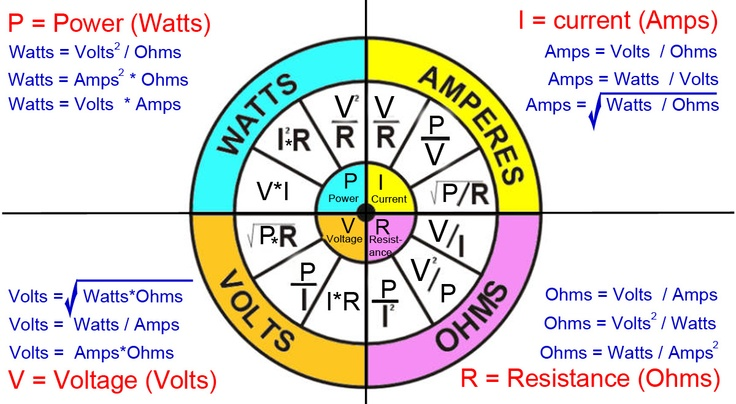Volt - unit of electrical potential or motive force - potential is required to send one ampere of current through one ohm of resistance

Ohm - unit of resistance - one ohm is the resistance offered to the passage of one ampere when impelled by one volt

Ampere - units of current - one ampere is the current which one volt can send through a resistance of one ohm

Watt - unit of electrical energy or power - one watt is the product of one ampere and one volt - one ampere of current flowing under the force of one volt gives one watt of energy

Volt-ampere (VA) - is a measurement of power in a direct current ( DC ) electrical circuit. The VA specification is also used in alternating current ( AC ) circuits, but it is less precise in this application, because it represents apparent power , which often differs from true power .

Kilovolt Ampere - one kilovolt ampere - KVA - is equal to 1000 volt amperes

Power Factor - ratio of watts to volt amperes

Most important Formulas:

Voltage V = I × R = P / I = √(P × R) in volts V

Current I = V / R = P / V = √(P / R) in amperes A

Resistance R = V / I = P / I2 = V2 / P in ohms Ω

Power P = V × I = R × I2 = V2 / R in watts WElectrical Potential - Ohm's Law

Ohm's law can be expressed as:

V = R I         (1a)

V = P / I         (1b)

V = (P R)1/2         (1c)

Electric Current - Ohm's Law

I = V / R         (2a)

I = P / V         (2b)

I = (P / R)1/2         (2c)

Electric Resistance - Ohm's Law

R = V / I         (3a)

R = V2/ P         (3b)

R = P / I2         (3c)

Electric Power

P = V I         (4a)

P = R I2         (4b)

P = V2/ R         (4c)

where

P = power (watts, W), V = voltage (volts, V)

I = current (amperes, A), R = resistance (ohms, Ω)

Electric Energy :Electric energy is power multiplied time, or

W = P t      (5)

where

W = energy (Ws, J), t = time (s)

Electrical Motors : Electrical Motor Efficiency

μ = 746 Php / Pinput_w         (6)

where

μ = efficiency

Php = output horsepower (hp)

Pinput_w = input electrical power (watts)

or alternatively

μ = 746 Php / (1.732 V I PF)         (6b)

Electrical Motor - Power

P3-phase = (V I PF 1.732) / 1,000         (7)

where

P3-phase = electrical power 3-phase motor (kW)

PF = power factor electrical motor

Electrical Motor - Amps

I3-phase = (746 Php) / (1.732 V μ PF)         (7)

where

I3-phase = electrical current 3-phase motor (amps)

PF = power factor electrical motor

Electrical measurements

 Quantity Name Definition frequency f hertz (Hz) 1/s force F newton (N) kg•m/s² pressure p pascal (Pa) = N/m² kg/m•s² energy E work joule (J) = N•m kg•m²/s² power P watt (W) = J/s kg•m²/s³ electric charge Q coulomb (C) = A•s A•s voltage V volt (V)= W/A kg•m²/A•s³ current I ampere (A) = Q/s A capacitance C farad (F) = C/V = A•s/V = s/Ω A²•s4/kg•m²